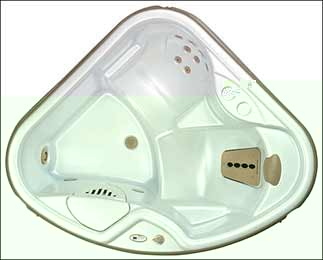2000-2005  Solana RX TX SX is indicated by the letter in the serial number. for example.

Solana TX:Serial Number  TX -3B2015 the serial number would read TX is model  3 third quarter B year(2000) and 2015 is the unit number made.

Serial Number  TX -3C2015 the serial number would read TX is model  3 third quarter C year(2001) and 2015 is the unit number made.

Serial Number  RX TX SX-3D2015 the serial number would read RX TX SX is model  3 third quarter D year(2002) and 2015 is the unit number made.

Serial Number  RX TX SX-3E2015 the serial number would read RX TX SX is model  3 third quarter E year(2003) and 2015 is the unit number made.

Serial Number  RX TX SX-3F2015 the serial number would read RX TX SX is model  3 third quarter F year(2004) and 2015 is the unit number made.

Serial Number  RX TX SX-3G2015 the serial number would read RX TX SX is model  3 third quarter G year(2005) and 2015 is the unit number made.

 Specifications Dimensions: 5’7" x 5’7" x 29" (1.7m x 1.7m x 0.74 m) Water Capacity: 130 gallons (492 liters) Weight: 316 lbs. (143 kg) dry 1,706 lbs. (774kg) filled Electrical Req: 115v, 15amp circuit 115v, 30amp circuit Ozonator: Not Available Filter: 71825 (Qty = 1)

Soalna SXSerial Number  TX -3B2015 the serial number would read TX is model  3 third quarter B year(2000) and 2015 is the unit number made.

Serial Number  TX -3C2015 the serial number would read TX is model  3 third quarter C year(2001) and 2015 is the unit number made.

Serial Number  RX TX SX-3D2015 the serial number would read RX TX SX is model  3 third quarter D year(2002) and 2015 is the unit number made.

Serial Number  RX TX SX-3E2015 the serial number would read RX TX SX is model  3 third quarter E year(2003) and 2015 is the unit number made.

Serial Number  RX TX SX-3F2015 the serial number would read RX TX SX is model  3 third quarter F year(2004) and 2015 is the unit number made.

Serial Number  RX TX SX-3G2015 the serial number would read RX TX SX is model  3 third quarter G year(2005) and 2015 is the unit number made.

 Specifications Dimensions: 6'6" x 6'6" x 33" (1.98m x 1.98m x .84m) Water Capacity: 285 gallons (1,079 liters) Weight: 439 lbs. (199 kg) dry 3,594 lbs. (1,630 kg) filled Electrical Req: 115v, 20amp circuit 115v, 30amp circuit Ozonator: FreshWater III Filter: 71825 (Qty = 1)

Solana RX:Serial Number  TX -3B2015 the serial number would read TX is model  3 third quarter B year(2000) and 2015 is the unit number made.

Serial Number  TX -3C2015 the serial number would read TX is model  3 third quarter C year(2001) and 2015 is the unit number made.

Serial Number  RX TX SX-3D2015 the serial number would read RX TX SX is model  3 third quarter D year(2002) and 2015 is the unit number made.

Serial Number  RX TX SX-3E2015 the serial number would read RX TX SX is model  3 third quarter E year(2003) and 2015 is the unit number made.

Serial Number  RX TX SX-3F2015 the serial number would read RX TX SX is model  3 third quarter F year(2004) and 2015 is the unit number made.

Serial Number  RX TX SX-3G2015 the serial number would read RX TX SX is model  3 third quarter G year(2005) and 2015 is the unit number made.

 Specifications Dimensions: 6’6" diameter x 33" (1.98 m x 0.84 m) Water Capacity: 245 gallons (927 liters) Weight: 345 lbs. (156 kg) dry 3,005 lbs. (1,363 kg) filled Electrical Req: 115v, 15amp circuit 115v, 30amp circuit Ozonator: Not Available Filter: 71825 (Qty = 1